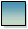### The number of hours left in a day is double the number of hours already passed. How many hours have already passed in the day?

8 hours

Step by Step Explanation:
1. According to the question,
number of hours left in the day = 2 × Number of hours already passed
2. Let the number of hours already passed be.
3. So, the number of hours left in the day =+4. We know that the total number of hours in a day = 24
So,++= 24
or,=
 24 3
= 8
5. Therefore, the number of hours already passed in the day = 8 hours